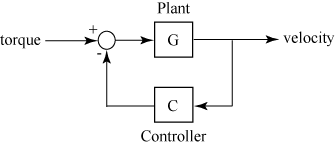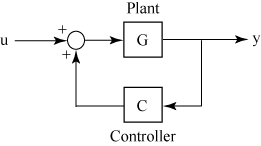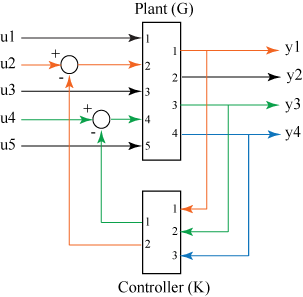# feedback

Feedback connection of multiple models

## Syntax

``sys = feedback(sys1,sys2)``
``sys = feedback(sys1,sys2,feedin,feedout)``
``sys = feedback(sys1,sys2,'name')``
``sys = feedback(___,sign)``

## Description

example

````sys = feedback(sys1,sys2)` returns a model object `sys` for the negative feedback interconnection of model objects `sys1,sys2`.From the figure, the closed-loop model `sys` has u as input vector and y as output vector. Both models, `sys1` and `sys2`, must either be continuous or discrete with identical sample times.```

example

````sys = feedback(sys1,sys2,feedin,feedout)` computes a closed-loop model `sys` using the input and output connections specified using `feedin` and `feedout`. Use this syntax when you want to connect only a subset of the available I/Os of MIMO systems.```

example

````sys = feedback(sys1,sys2,'name')` computes a closed-loop model `sys` with the feedback connections specified by the respective I/O names of MIMO models `sys1` and `sys2`. Use the `'name'` flag only when all the required I/Os in the set of MIMO systems are properly named.```

example

````sys = feedback(___,sign)` returns a model object `sys` for a feedback loop with the type of feedback specified by `sign`. By default, `feedback` assumes negative feedback and is equivalent to `feedback(sys1,sys2,-1)`. To compute the closed-loop system with positive feedback, use ```sign = +1```.```

## Examples

collapse all

`pendulumModelAndController.mat` contains a SISO inverted pendulum transfer function model `G` and its associated PID controller `C`.Load the inverted pendulum and controller model to the workspace.

```load('pendulumModelAndController','G','C'); size(G)```
```Transfer function with 1 outputs and 1 inputs. ```
`size(C)`
```PID controller with 1 output and 1 input. ```

Use `feedback` to create the negative feedback loop with `G` and `C`.

`sys = feedback(G*C,1)`
```sys = 1.307e-06 s^3 + 3.136e-05 s^2 + 5.227e-06 s --------------------------------------------------------- 2.3e-06 s^4 + 1.725e-06 s^3 - 4.035e-05 s^2 - 5.018e-06 s Continuous-time transfer function. ```

`sys` is the resultant closed loop continuous-time transfer function obtained using negative feedback. `feedback` converts the PID controller model C to a transfer function before connecting it to the continuous-time transfer function model `G`. For more information, see Rules That Determine Model Type.

For this example, consider two transfer functions that describe a plant `G` and controller `C` respectively.`$G\left(s\right)=\frac{2{s}^{2}+5s+1}{{s}^{2}+2s+3}\phantom{\rule{0.2777777777777778em}{0ex}}\phantom{\rule{0.2777777777777778em}{0ex}}\phantom{\rule{0.2777777777777778em}{0ex}}\phantom{\rule{0.2777777777777778em}{0ex}}\phantom{\rule{0.2777777777777778em}{0ex}}\phantom{\rule{0.2777777777777778em}{0ex}}C\left(s\right)=\frac{5\left(s+2\right)}{s+10}$`

Create the plant and controller transfer functions.

```G = tf([2 5 1],[1 2 3],'inputname',"torque",'outputname',"velocity"); C = tf([5,10],[1,10]); ```

Use `feedback` to create the negative feedback loop using `G` and `C`.

`sys = feedback(G,C,-1)`
```sys = From input "torque" to output "velocity": 2 s^3 + 25 s^2 + 51 s + 10 --------------------------- 11 s^3 + 57 s^2 + 78 s + 40 Continuous-time transfer function. ```

`sys` is the resultant closed loop transfer function obtained using negative feedback with torque as the input and velocity as the output.

For this example, consider two transfer functions that describe a plant `G` and controller `C` respectively.`$G\left(s\right)=\frac{2{s}^{2}+5s+1}{{s}^{2}+2s+3}\phantom{\rule{0.2777777777777778em}{0ex}}\phantom{\rule{0.2777777777777778em}{0ex}}\phantom{\rule{0.2777777777777778em}{0ex}}\phantom{\rule{0.2777777777777778em}{0ex}}\phantom{\rule{0.2777777777777778em}{0ex}}\phantom{\rule{0.2777777777777778em}{0ex}}C\left(s\right)=\frac{5\left(s+2\right)}{s+10}$`

Create the plant and controller transfer functions.

```G = tf([2 5 1],[1 2 3],'inputname',"torque",'outputname',"velocity"); C = tf([5,10],[1,10]); ```

Use `feedback` to create the positive feedback loop using `G` and `C`.

`sys = feedback(G,C,+1)`
```sys = From input "torque" to output "velocity": -2 s^3 - 25 s^2 - 51 s - 10 --------------------------- 9 s^3 + 33 s^2 + 32 s - 20 Continuous-time transfer function. ```

`sys` is the resultant closed loop transfer function obtained using positive feedback with torque as the input and velocity as the output.

Based on the figure below, consider connecting two MIMO transfer functions with two inputs and two outputs in a negative feedback loop.For this example, create two random continuous state-space models using `rss`.

```G = rss(4,2,2); C = rss(2,2,2); size(G)```
```State-space model with 2 outputs, 2 inputs, and 4 states. ```
`size(C)`
```State-space model with 2 outputs, 2 inputs, and 2 states. ```

Use `feedback` to connect the two state-space models in a negative feedback loop according to the above figure.

```sys = feedback(G,C,-1); size(sys)```
```State-space model with 2 outputs, 2 inputs, and 6 states. ```

The resulting state-space model `sys` is a 2 input, 2 output model with 6 states. The negative feedback loop is completed such that,

• The first output of `G` is connected to the first input of `C`

• The second output of `G` is connected to the second input of `C`

`mimoPlantAndController.mat` contains a 2 input, 2 output transfer function plant model `G` and a 2 input, 2 output transfer function controller model `C` to be connected as follows:First, load the plant and controller models to the workspace.

```load('mimoPlantAndController.mat','G','C'); size(G)```
```Transfer function with 2 outputs and 2 inputs. ```
`size(C)`
```Transfer function with 2 outputs and 2 inputs. ```

By default, `feedback` would connect the first output of `G` to the first input of `C` and the second output of `G` to the second input of `C`. In order to connect the plant and controller according to the figure, name the respective I/Os of the two systems to ensure the correct connections.

`G.InputName `
```ans = 2x1 cell {'torque'} {'angle' } ```
`G.OutputName`
```ans = 2x1 cell {'velocity'} {'force' } ```
`C.InputName`
```ans = 2x1 cell {'force' } {'velocity'} ```
`C.OutputName`
```ans = 2x1 cell {'angle' } {'torque'} ```

Then use the `'name'` flag with the `feedback` command to make the connections according to the I/O names.

`sys = feedback(G,C,'name');`

The resulting closed loop negative feedback transfer function `sys` has the feedback connections in the required order.

Consider a state-space plant `G` with five inputs and four outputs and a state-space feedback controller `K` with three inputs and two outputs. The outputs 1, 3, and 4 of the plant `G` must be connected the controller `K` inputs, and the controller outputs to inputs 2 and 4 of the plant.For this example, generate randomized continuous-time state-space models using `rss` for both `G` and `K`.

```G = rss(3,4,5); K = rss(3,2,3);```

Define the `feedout` and `feedin` vectors based on the inputs and outputs to be connected in a feedback loop.

```feedin = [2 4]; feedout = [1 3 4]; sys = feedback(G,K,feedin,feedout,-1); size(sys)```
```State-space model with 4 outputs, 5 inputs, and 6 states. ```

`sys` is the resultant closed loop state-space model obtained by connecting the specified inputs and outputs of `G` and `K`.

## Input Arguments

collapse all

Systems to connect in a feedback loop, specified as dynamic system models. Dynamic systems that you can use include:

When `sys1` and `sys2` are two different model types, `feedback` uses precedence rules to determine the resulting model `sys`. For example, when a state-space model and a transfer function is connected in a feedback loop, the resulting system is a state-space model based on the precedence rules. For more information, see Rules That Determine Model Type.

Subset of inputs to be used, specified as a vector.From the figure, `feedin` contains indices of the input vector of MIMO plant `P` and specifies which subset of inputs u are involved in the feedback loop. The resulting model `sys` has the same inputs as `G`, with their orders preserved.

For an example, see Specify Input and Output Connections in a Feedback Loop.

Subset of outputs to be used, specified as a vector.

`feedout` specifies which outputs of MIMO plant `G` are used for feedback. The resulting model `sys` has the same outputs as `G`, with their orders preserved.

For an example, see Specify Input and Output Connections in a Feedback Loop.

Type of feedback, specified as `-1` for negative feedback or `+1` for positive feedback. `feedback` assumes negative feedback by default.

## Output Arguments

collapse all

Closed-loop system, returned as a SISO, or MIMO dynamic system model. `sys` can be one of the following depending on the precedence rules:

When `sys1` and `sys2` are two different model types, `feedback` uses precedence rules to determine the resulting model `sys`. For example, when a state-space model and a transfer function is connected in a feedback loop, the resulting system is a state-space model based on the precedence rules outlined in Rules That Determine Model Type.

## Limitations

• The feedback connection must be free of algebraic loops. For instance, if D1 and D2 are the feedthrough matrices of `sys1` and `sys2`, this condition is equivalent to:

• I + D1D2 nonsingular when using negative feedback

• I − D1D2 nonsingular when using positive feedback